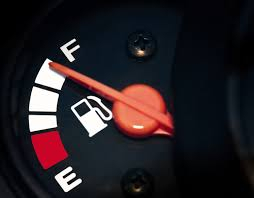# Car fuel

Tanya drove 3 1/2 hours and used 1/3 tank of gas. For how long could Tanya drive using a 1/2 tank of gas?

Enter your answer as a mixed number in the simplest form in the box.

t2 =  5 14 = 21/4 h

### Step-by-step explanation:Did you find an error or inaccuracy? Feel free to write us. Thank you!

Tips for related online calculators
Need help calculating sum, simplifying, or multiplying fractions? Try our fraction calculator.
Need help with mixed numbers? Try our mixed-number calculator.
Check out our ratio calculator.
Tip: Our volume units converter will help you convert volume units.
Do you want to convert time units like minutes to seconds?